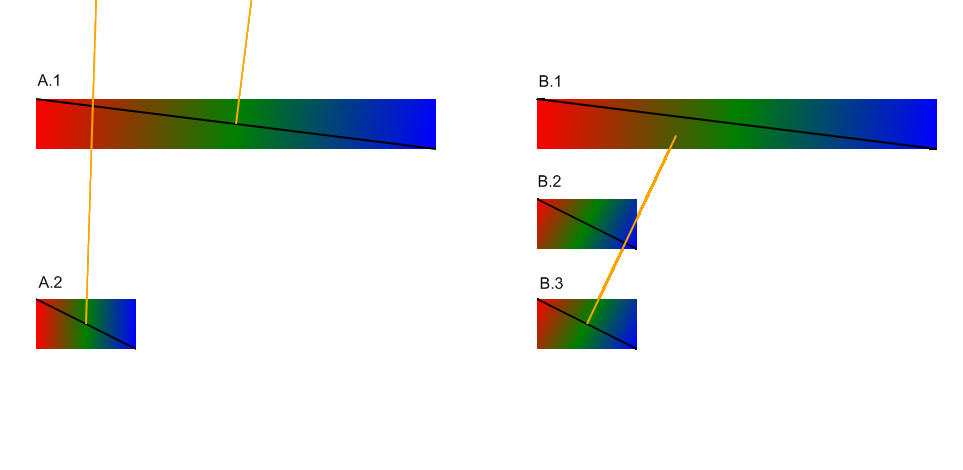# Re: Fwd: Question on gradient userSpaceOnUse

From: Anthony Grasso <anthony.grasso@cisra.canon.com.au>
Date: Thu, 07 Jan 2010 23:19:37 +1100
Message-ID: <4B45D159.6010302@cisra.canon.com.au>
CC: www-svg <www-svg@w3.org>
```Hi Jeff,

I spent some time a while back tackling this problem. See comments below.

Jeff Schiller wrote:
> Hi www-svg,
>
> This is an email I forwarded to Erik Dahlstrom to question about Opera
> behavior, but thought it appropriate for general SVG WG discussion.
> Consider the following sample:
>
>
> <?xml version="1.0"?>
> <svg xmlns="http://www.w3.org/2000/svg"
> <defs>
>  <linearGradient id="g1" x1="0" y1="0" x2="400" y2="50"
>    <stop offset="0" stop-color="red"/>
>    <stop offset="0.5" stop-color="green"/>
>    <stop offset="1.0" stop-color="blue"/>
>  <linearGradient id="g2" x1="0" y1="100" x2="100" y2="150"
>    <stop offset="0" stop-color="red"/>
>    <stop offset="0.5" stop-color="green"/>
>    <stop offset="1.0" stop-color="blue"/>
> </defs>
>
> <g transform="translate(100,100)">
>  <rect width="400" height="50" fill="url(#g1)" transform="scale(0.25,1)"/>
>  <rect y="100" width="100" height="50" fill="url(#g2)" />
> </g>
>
> </svg>
>
> In the above test, Firefox, WebKit and Batik show the top gradient as
> nearly horizontal while Opera shows the two gradients identically.
>
> The spec says userSpaceOnUse "shall represent values in the coordinate
> system that results from taking the current user coordinate system in
> place at the time when the gradient element is referenced".
>
> Can someone please clarify what is the correct behavior here?
>

I think the wording below  is very ambiguous.

[[
If gradientUnits="userSpaceOnUse", 'x1', 'y1', 'x2', 'y2' shall represent values
in the coordinate system that results from taking the current user coordinate
system in place at the time when the gradient element is referenced"
]]

The diagrams that Ken and Cyril presented illustrate one vital detail required
for rendering linear gradients which is not present in any of the SVG
specifications. A gradient direction is defined by the vector, but there is a
second component which defines the direction of the stripes on a linear gradient
- the Gradient Normal. Ken's and Cyril's diagrams both show the Gradient Normal.

The problem is at what point in time the gradient normal is transformed. From a
technical perspective the two different results are correct (see
both_sides_of_the_coin.png).

Implementation 'A' defines a Gradient Vector and a Gradient Normal prior to
being transformed (A.1). When the transformation is applied both vector and
normal are transformed (A.2).

Implementation 'B' defines a Gradient Vector only prior to being transformed
(B.1). When the transform is applied the vector is transformed (B.2). The normal
is then defined on the gradient (B.3) prior to any gradientTransform attributes
being applied.

Given that the specification is ambiguous about the 'time' a gradient element is
reference and additionally does not define how a gradient normal works with a
gradient vector both implementations are technically correct. The question then
remains which looks better (see real_world_01.png and real_world_02.png).

This is something that will must be made clear for SVG 2.0, hence an issue
should be raised.

Cheers,

Anthony



```(image/png attachment: both_sides_of_the_coin.png)(image/png attachment: real_world_01.png)(image/png attachment: real_world_02.png)

Received on Thursday, 7 January 2010 12:20:21 UTC

This archive was generated by hypermail 2.3.1 : Wednesday, 8 March 2017 09:47:19 UTC